## RS Aggarwal Class 8 Solutions Chapter 6 Operations on Algebraic Expressions Ex 6A

These Solutions are part of RS Aggarwal Solutions Class 8. Here we have given RS Aggarwal Solutions Class 8 Chapter 6 Operations on Algebraic Expressions Ex 6A.

Other Exercises

Question 1.
Solution:
8ab + ( – 5ab) + (3ab) + ( – ab)
= 8ab – 5ab + 3ab – ab = 11 ab – 6ab
= 5ab Ans.

Question 2.
Solution:
7x + ( – 3x) + 5x + ( – x) + ( – 2x)
= 7x – 3x + 5x – x – 2x
= 12x – 6x = 6x Ans.

Question 3.
Solution: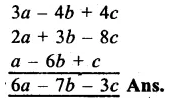Question 4.
Solution: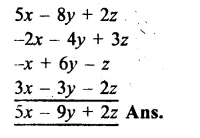Question 5.
Solution:Question 6.
Solution: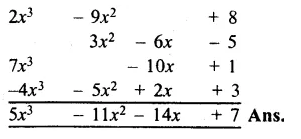Question 7.
Solution:Question 8.
Solution: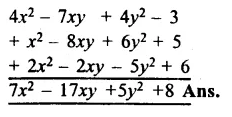Subtarct:

Question 9.
Solution:
-5a²b – 3a²b = – 8a²b Ans.

Question 10.
Solution:
6pq – ( – 8pq) = 6pq + 8pq

Question 11.
Solution:
– 8abc – ( – 2abc)
= – 8abc + 2abc
= – 6abc Ans.

Question 12.
Solution:
– 11p – ( – 16p)
= – 11p + 16p
= 5p Ans.

Question 13.
Solution: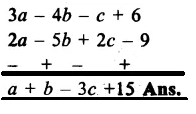Question 14.
Solution: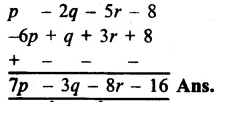Question 15.
Solution: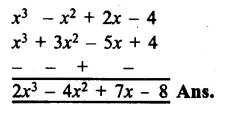Question 16.
Solution:Question 17.
Solution: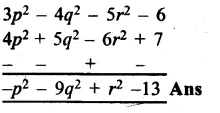Question 18.
Solution: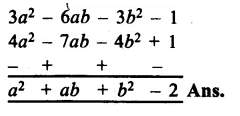Question 19.
Solution:
Let length of rectangle = 5x2 – 3y2
and breadth = x2 + 2xy
= 2(5x2 – 3y2 + x2 + 2xy)
= 2(6x2 – 3y2 + 2xy)
= 12x2 – 6y2 + 4xy Ans.

Question 20.
Solution:
Perimeter of a triangle = 6p2 – 4p + 9
Sum of two sides of it = 3p2 – 5p + 3 + p2 – 2p + 1 = 4p2 – 7p – 4
Third side = (6p2 – 4p + 9) – (4p2 – 7p + 4)
= 6p2 – 4p + 9 – 4p2 + 7p – 4
= 2p2 + 3p + 5 Ans

Hope given RS Aggarwal Solutions Class 8 Chapter 6 Operations on Algebraic Expressions Ex 6A are helpful to complete your math homework.

If you have any doubts, please comment below. Learn Insta try to provide online math tutoring for you.